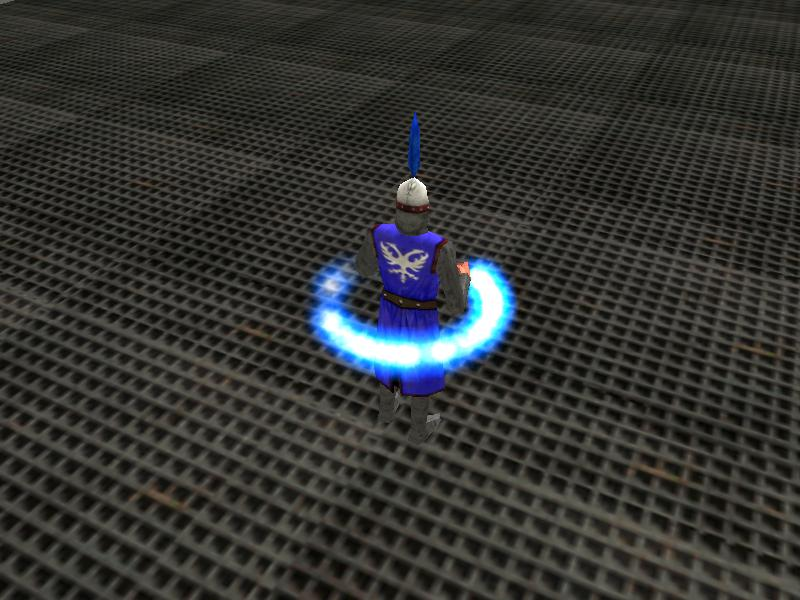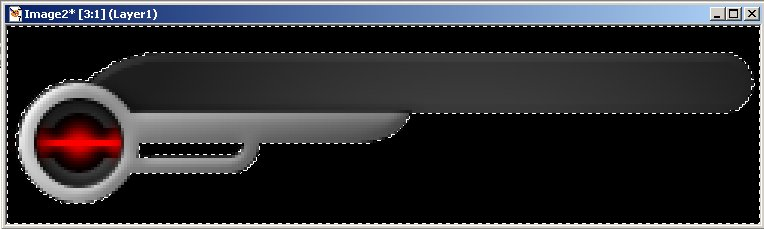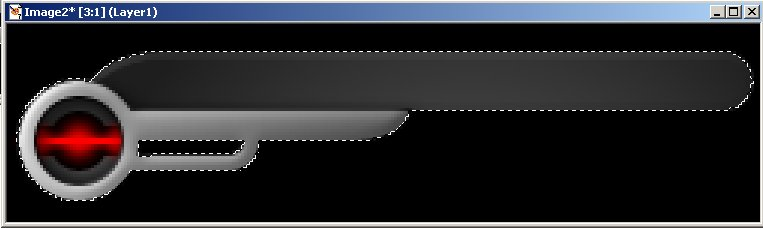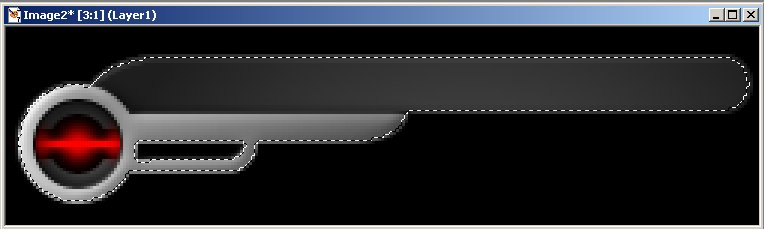Questions from the forum
 Q: How can I get a function to spawn particles in a circle around my character? A: Here's an example.   BMAP px_tga = "px.tga";   function fade_p() {        my.alpha -= 10 * time_step;        if (my.alpha < 0) {my.lifespan = 0;} }   function effect_x() {        my.alpha = 30 + random(65);        my.flare = on;        my.bmap = px_tga;        my.size = 12;        my.bright = on;        my.move = on;        my.function = fade_p; }   function create_px() {        var temp_pos;        var temp_var;        while (1)        {                temp_var += 35 * time_step; // 35 = particle rotation speed                vec_set (temp_pos.x, my.x);                temp_pos.x += 20 * cos(temp_var); // 20 = particle radius                temp_pos.y += 20 * sin(temp_var); // use the same value here                temp_pos.z += 10; // play with 10                effect(effect_x, 1, temp_pos.x, nullvector);                wait (1);        } }   action players_code() // simple player code {        create_px();        player = my; // I'm the player        while (1)        {                // move the player using the "W", "S", "A" and "D" keys; "10" = movement speed, "6" = strafing speed                c_move (my, vector(10 * (key_w - key_s) * time_step, 6 * (key_a - key_d) * time_step, 0), nullvector, glide);                wait (1);        } }Q: How can I fade out a sound that is played by snd_loop? A: Use this example as a base for your code.   var loop_handle; var loop_volume = 100;   sound atmosphere_wav = "atmosphere.wav";   function loop_startup() {        loop_handle = snd_loop(atmosphere_wav, 80, 0);        while (!key_f) {wait (1);}        while (loop_volume > 1)        {                snd_tune(loop_handle, loop_volume, 0, 0);                loop_volume -= 0.5 * time_step; // 0.5 = fading speed                wait (1);        }        snd_stop (loop_handle); }     Q: I need a 3D "button" which gets colored to red when it is clicked and returns to its normal color after the second click. A: Use this piece of code.   BMAP arrow_pcx = "arrow.pcx";   function mouse_startup() {         mouse_mode = 2;        mouse_map = arrow_pcx;        while (1)        {                 vec_set(mouse_pos, mouse_cursor);                wait(1);        } }   var clicked_times = 0;   function light_me_up() {        clicked_times += 1;        my.lightred = 255 * (clicked_times % 2); }   action button_3d {        my.light = on;        my.enable_click = on;        my.event = light_me_up; }     Q: I have created some pcx panels which use the overlay flag. When I run it the project, my panels still have some residual black spots around them. Has anyone found a fix to this? A: You have to turn your pcx files into tga bitmaps. Here's a quick example that uses Paint Shop Pro   - Open your pcx file, and then use the magic wand to select the black areas of the panel that aren't useful.- Invert the selection.- Contract the selection by 1 or more pixels, as needed (Selections -> Modify -> Contract in Paint Shop Pro)- Save the resulting selection to the alpha channel (Selections -> Save to alpha channel in Paint Shop Pro) That's all! Save the bitmap as a tga file and you'll be able to use your panel without seeing any of the black edges.     Q: How can I change the X position of a model using the keyboard? A: Attach this action to your player.   action players_code() // simple player and camera code {        var anim_percentage;        player = my; // I'm the player        camera.pan = 90;        while (1)        {                // move the player using the left and right arrow keys                c_move (my, vector(10 * (key_cur - key_cul) * time_step, 0, 0), nullvector, glide); // 10 = speed                if (key_cur || key_cul) // one of the movement keys is pressed?                {                        ent_animate(my, "walk", anim_percentage, anm_cycle);                        anim_percentage += 8 * time_step; // "8" controls the "walk" animation speed                }                else // the player is standing still?                {                        ent_animate(my, "stand", anim_percentage, anm_cycle);                        anim_percentage += 1 * time_step; // "1" controls the "stand" animation speed                }                vec_set (camera.x, player.x);                camera.y -= 300; // place the camera 300 quants behind the player (play with this value)                camera.z += 40; // place the camera 40 quants above the player on the z axis (play with this value)                wait (1);        } }     Q: I have an entity that should display a new string above its head every 3 seconds, reading it from a text file. Can anyone help? A: Use the example below; it displays all the lines inside the talk.txt file for as long as the entity is visible on the screen.   var eof_reached = 0; // will be set to -1 when the end of the file (eof) is reached var string_handle;   STRING talker_string = "                                        "; // the string can store up to 40 characters   TEXT talker_txt = {        pos_x = 300;        pos_y = 200;        string (talker_string);        flags = VISIBLE; }   action talking_entity() {        var text_position;        var temp_pos;        var n = 0;        string_handle = file_open_read("talk.txt"); // put your lines of text inside this file        while (1)        {                vec_set (text_position, my.x);                text_position.z += 40; // play with this value                vec_set (temp_pos.x, my.x);                if (vec_to_screen(temp_pos.x, camera)) // if the entity is visible on the screen                {                        talker_txt.visible = ON;                        if (eof_reached != -1) // the end of the file wasn't reached yet?                        {                                eof_reached = file_str_read(string_handle, talker_string); // then read a string from the file                                n += 1; // move on to the following string                                sleep (3); // read a new string from the file every 3 seconds                        }                        else                        {                                file_close (string_handle); // all the strings are read here, so close the file                        }                }                else // the entity isn't visible on the screen?                {                        talker_txt.visible = OFF;                }                wait (1);        }                 }     Q: My model includes an animation named "dance". How can I call this animation when the player hits something, then restore it to "walk" when the "dance" frames end? A: Here's an example that takes care of the dancing, walking and standing.   var dancing = 0;   function do_the_dance() {        var dance_percentage = 0;        dancing = 1;        while (dance_percentage < 100)        {                ent_animate(my, "dance", dance_percentage, anm_cycle);                dance_percentage += 2 * time_step; // "2" controls the "dance" animation speed                wait (1);        }        dancing = 0; }   action players_code() {        var anim_percentage;        player = my; // I'm the player        camera.pan = 90;        my.enable_impact = on;        my.enable_entity = on;        my.enable_block = on;        my.event = do_the_dance;        while (1)        {                if (dancing == 0)                {                        // move the player using the "W", "S", "A" and "D" keys; "10" = movement speed, "6" = strafing speed                        c_move (my, vector(10 * (key_w - key_s) * time_step, 6 * (key_a - key_d) * time_step, 0), nullvector, glide);                        if (key_w || key_s || key_a || key_d)                        {                                ent_animate(my, "walk", anim_percentage, anm_cycle);                                anim_percentage += 8 * time_step; // "8" controls the "walk" animation speed                        }                        else // the player is standing still?                        {                                ent_animate(my, "stand", anim_percentage, anm_cycle);                                anim_percentage += 1 * time_step; // "1" controls the "stand" animation speed                        }                }                wait (1);        } }     Q: I want to display a question on the screen, and then to accept the answer and compare it with the predefined answer in the code. If it is correct, then the code should display the next question and accept the answer, and so on. A: There you go.   STRING answer1_str = "                              "; // stores up to 30 characters STRING answer2_str = "                              "; // stores up to 30 characters   TEXT question1_txt = {        layer = 15;        pos_x = 250;        pos_y = 50;        string ("2 + 2 = ?"); }   TEXT answer1_txt = {        layer = 15;        pos_x = 330;        pos_y = 50;        string (answer1_str); }   TEXT question2_txt = {        layer = 15;        pos_x = 250;        pos_y = 50;        string ("3 + 3 = ?"); }   TEXT answer2_txt = {        layer = 15;        pos_x = 330;        pos_y = 50;        string (answer2_str); }   function answers_startup() {        sleep (2);        question1_txt.visible = ON;        while (1)        {                str_cpy(answer1_str, ""); // reset the string                answer1_txt.visible = ON;                inkey(answer1_str);                if (str_cmpi(answer1_str, "4"))                {                        str_cpy(answer1_str, "Good job!");                        sleep (2);                        question1_txt.visible = OFF;                        answer1_txt.visible = OFF;                        break;  // Got the right answer? The move on to the following question!                }                else                {                        str_cpy(answer1_str, "Wrong! Please try again!");                        sleep (3);                }                wait (1);        }        question2_txt.visible = ON;        while (1)        {                str_cpy(answer2_str, ""); // reset the string                answer2_txt.visible = ON;                inkey(answer2_str);                if (str_cmpi(answer2_str, "6"))                {                        str_cpy(answer2_str, "Good job!");                        sleep (2);                        question1_txt.visible = OFF;                        answer1_txt.visible = OFF;                        break;  // Got the right answer? The move on to the following question!                }                else                {                        str_cpy(answer2_str, "Wrong! Please try again!");                        sleep (3);                }                wait (1);        }        // your 3rd question would go here, etc        sys_exit(NULL); // shut down the engine }     Q: I'd like to have a turret who can detect friends and foes. If a friendly entity comes near the turret, the turret should just follow it. On the other hand, if the player approaches, the turret should rotate towards it and fire at it. A: Use this snippet.   SOUND destroyed_wav = "destroyed.wav";   STRING explo13_pcx = "explo+13.pcx"; STRING rocket_mdl = "rocket.mdl";   action my_player() {        my.enable_scan = ON;        player = my; // I'm the player        ............... // the rest of your player code would go here }   function explode_rocket() {        var anim_speed;        anim_speed = 0.7 + random(1.3);        my.light = on;        my.lightrange = 0;        my.red = 55 + random(200);        my.green = random(255);        my.blue = 0;        my.nofog = on;        my.oriented = on;        my.flare = on;        my.bright = on;        my.ambient = random(100);        my.passable = on;        my.roll = random(360); // pick a random orientation        my.skill30 = you.skill10; // store skill10 quickly because "you" will be removed soon        while (my.frame < 14)        {                if (my.scale_x < my.skill30) // grow until you reach the value that was stored or set in skill10                {                        my.scale_x += 5 * time_step;                        my.scale_y = my.scale_x;                }                my.frame += anim_speed * time;                  vec_set (temp.x, camera.x);                vec_sub (temp.x, my.x);                vec_to_angle (my.pan, temp); // turn towards the player to make sure that the explo looks great                  wait (1);        }        ent_remove (me); }   function remove_rocket() {        my.passable = ON;        wait (1);        my.event = NULL;        ent_playsound (my, destroyed_wav, 1000); // play the explosion sound        ent_create (explo13_pcx, my.pos, explode_rocket);        my.invisible = on; // hide the rocket, keep ent_playsound playing        sleep (2); // for 2 more seconds        ent_remove(me); // now remove it }   function move_rocket() {        my.pan = you.pan;        my.tilt = you.tilt;        wait (1);        var rocket_speed;        var tempo_pos;        my.enable_entity = on;        my.enable_impact = on;        my.enable_block = on;        my.event = remove_rocket;        my.skill40 = 1234; // I'm a rocket        my.skill20 = 0;        my.skill10 = 3; // default explosion scale for the rocket        while (my.skill20 < 5000)        {                my.roll += 50 * time_step;                rocket_speed.x = 200 * time_step;                rocket_speed.y = 0;                rocket_speed.z = 0;                my.skill20 += 1 * time_step;                c_move (my, rocket_speed, nullvector, IGNORE_PASSABLE);                wait (1);        }        remove_rocket(); }   action turret() {        var bullet_offset;        var temp_turret;        while (1)        {                c_scan(my.x, my.pan, vector(360, 180, 2000), IGNORE_ME | SCAN_ENTS);                if (you) // an entity was detected by c_scan?                {                        vec_set (temp_turret.x, you.x);                        vec_sub (temp_turret.x, my.x);                        vec_to_angle (my.pan, temp_turret); // then rotate the turret towards the entity that was detected                }                if (you == player) // detected the player?                {                        if (vec_dist (my.x, player.x) < 700) // the turret starts to fire if the player comes closer than 700 quants                        {                                bullet_offset.x = 100;                                bullet_offset.y = 0;                                bullet_offset.z = 0;                                vec_rotate (bullet_offset, my.pan);                                vec_add (bullet_offset.x, my.x);                                ent_create (rocket_mdl, bullet_offset.x, move_rocket);                                sleep (2); // fires a bullet every 2 seconds                        }                }                wait (1);        } }   Q: I want to fill a test-tube with a given liquid. During runtime the test-tube has to be filled using mouse press or using arrows on the keyboard. A: Use several "window" definitions and this piece of code as an example.   var red_substance = 0;   BMAP arrow_pcx = "arrow.pcx";   function mouse_startup() {         mouse_mode = 2;        mouse_map = arrow_pcx;        while (1)        {                 vec_set(mouse_pos, mouse_cursor);                wait(1);        } }   function subtract_red() {        while (mouse_left)        {                red_substance -= 25 * time_step;                wait (1);        } }   function add_red() {        while (mouse_left)        {                red_substance += 25 * time_step;                wait (1);        } }   PANEL red_pan = {        bmap = "red.pcx";        window (7, 4, 81, 477, "fluidred.pcx", 0, red_substance);        button (25, 480, "addh.pcx", "addl.pcx", "addh.pcx", add_red, NULL, NULL);        button (60, 480, "subh.pcx", "subl.pcx", "subh.pcx", subtract_red, NULL, NULL);        flags = VISIBLE; }